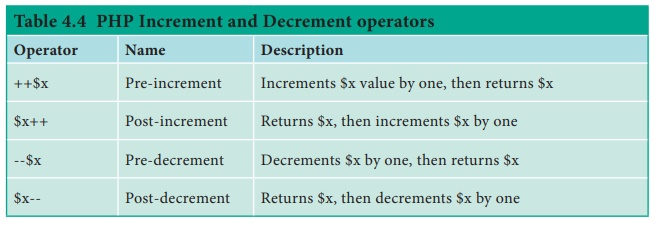Home | | Computer Application 12th Std | Operators in PHP

# Operators in PHP

Operator is a symbol which is used to perform mathematical and logical operations in the programing languages.

Operators in PHP

Operator is a symbol which is used to perform mathematical and logical operations in the programing languages. Different types of operator in PHP are:

1. Arithmetic operators,

2. Assignment operators,

3. Comparison operators,

4. Increment/Decrement operators,

5. Logical operators, and

6. String operators.

### 1. Arithmetic operators

The arithmetic operators in PHP perform general arithmetical operations, such as addition, subtraction, multiplication and division etc. Refer Table 4.1.### 2. Assignment Operators:

Assignment operators are performed with numeric values to store a value to a variable. The default assignment operator is “=”. This operator sets the left side operant value of expression to right side variable. Refer Table 4.2.### 3. Comparison Operators:

Comparison operators perform an action to compare two values. These values may contain integer or string data types (Number or Strings). Refer Table 4.3.### 4. Increment and Decrement Operators:

Increment and decrement operators are used to perform the task of increasing or decreasing variable’s value. This operator is mostly used during iterations in the program logics. Refer Table 4.4.### 5. Logical Operators:

Logical Operators are used to combine conditional statements. Refer Table 4.5.### 6. String Operators:

Two operators are used to perform string related operations such as Concatenation and Concatenation assignment (Appends). Refer Table 4.6.Tags : PHP (Hypertext Pre-Processor) , 12th Computer Applications : Chapter 4 : Introduction to Hypertext Pre-Processor (PHP)
Study Material, Lecturing Notes, Assignment, Reference, Wiki description explanation, brief detail
12th Computer Applications : Chapter 4 : Introduction to Hypertext Pre-Processor (PHP) : Operators in PHP | PHP (Hypertext Pre-Processor)